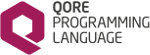Qore Programming Language Reference Manual  0.9.16
References

# Overview of References

References allow for addressing other lvalues, including complex lvalue expressions, with an alias called a reference. References to lvalues are defined by placing a `"\"` character in front of an expression that gives an lvalue, such as in the following examples:

reference r1 = \a;
reference r2 = \recs[rn].order[on];

References are especially convenient when aliasing an internal part of a complex data structure. Consider the following example (a list of records, where each record is a hash with a list of orders under the `"order"` key - in the following example, we want to set the last record's last order's `"numberofitems"` key to be equal to the number of elements under the order's `"items"` key):

recs[recs.size() - 1].order[recs[recs.size() - 1].order.size() - 1].numberofitems = recs[recs.size() - 1].order[recs[recs.size() - 1].order.size() - 1].items.size();

In the above code, using references could greatly simplify the readability of the code:

reference lastrec = \recs[recs.size() - 1];
reference lastorder = \lastrec.order[lastrec.order.size() - 1];
lastorder.numberofitems = lastorder.items.size();
Note
Local variables that are referenced are automatically converted to a special type of local variable that is protected by a mutual-exclusion thread lock so that the reference can be safely used in background threads. The lvalue represented by the local variable will exist beyond its local scope; as long as the reference to the lvalue exists, the local variable's lvalue will exist and remain valid.
Since
Qore 0.8.5 universal lvalue references are supported; previously references could only be used with arguments to a function or method call or the like. As of Qore 0.8.5+, references can be used anywhere in Qore in any expression, even with references to local variables in background expressions and in closure argument lists.

# Reference Type Restrictions

The following reference type restrictions exist:

Once assigned, a reference is permanently assigned to the lvalue; there is currently no way to "unreference" a reference.

The *reference has special behavior when used as an argument type restriction. In this case, if no value is assigned to the reference in the call, the local variable can be assigned to any value in the code block, even if the called did not pass a value to the reference. This allows variables of "*reference" type to be used even without a reference assigned.

Consider the following example:

sub ref(*reference r) {
r = {};
}
hash h;
# in this case, the reference in "ref()" updates the local variable in "ref()"
ref();
# in this case, the reference updates top-level local variable "h"
ref(\h);

The above code is valid and will work as described in the comments above.